# ICML and isotropic position of a convex body

ICML 2013 just finished a few days ago. The presentation that inspired me the most was the invited talk by Santosh Vempala. He talked about the relations between sampling, optimization, integration, learning, and rounding. I strongly recommend Vempala’s short survey on these topics, it is an excellent read. I also would like to point out two papers by Vempala on optimization that are very interesting and exceptionally well written: the first paper (with Bertsimas) presents an optimal (in terms of oracle complexity) algorithm for convex optimization with a much better computational complexity than the Center of Gravity method (but still slightly worse computational complexity than the Ellipsoid Method); the second paper (with Kalai) gives a more efficient algorithm than the Ellipsoid Method for linear optimization with a membership oracle (instead of a separation oracle).

Vempala’s talk gave me the motivation to write on a related topic which has many applications in optimization, probability and statistics: this is the notion of an isotropic convex body. A convex bodyis centered and isotropic if it satisfies: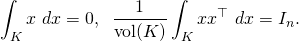First observe that the hypercube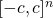is isotropic (withof order), and it is a one line calculation to show that(with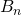being the Euclidean ball of unit radius andsome numerical constant) is also isotropic. It is also immediate that any convex body can be put in isotropic position up to an affine transformation. Interestingly it was shown in this paper that one can find an approximate isotropic transformation with a linear (in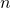) number of samples from the uniform distribution on.

If you read papers about isotropic convex set they often use a definition where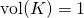, in which case the second moment condition writes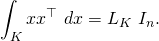The termis called the isotropic constant. However this definition of isotropy is not easy to work with because there is a deep conjecture related to the order of magnitude of the isotropic constant. It is easy to see thathas to be at least some numerical constant, but the statement thatis also upper bounded by a numerical constant is equivalent to the Hyperplane Conjecture (which I describe below). For this reason we stick to the definition with the second moment normalization.

The question we want to explore now is the following: ‘what looks like an isotropic convex body‘? We are especially interested in the answer for the high-dimensional regime whereis very large. The following three results give interesting and useful results in that direction. I useto denote some numerical constant.

KLS lemmaIt can be shown that the above inequalities are both tight in the simplex case.

We describe the next results in terms of a random variable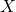uniformly distributed on. Sinceis isotropic one has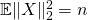. The next result shows that in facthas norm of orderwith high probability.

Theorem (Paouris’ inequality)

For any,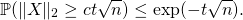The next statement is still a conjecture, it concerns a much more refined concentration inequality for the norm of an isotropic random variable.

Thin Shell Conjecture

For any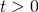,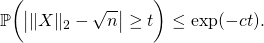Since we are now talking about conjectures I cannot resist giving you the most important conjecture in convex geometry:

Hyperplane Conjecture

For any centered isotropicthere exists an hyperplane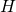such thatAs of today the best result we know replacesbyin the above inequality (this is a theorem of Bourgain up to a log factor, and the log factor was removed by Klartag). To the unexperienced eye as mine it seems unbelievable that this is still a conjecture: it looks clearly true! The following theorem reminds us that the set of statements that look true is not equal to the set of true statements…

Theorem (Busemann-Petty problem)

Ifthere exists centered convex setsand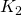such that for all hyperplanesone hasandThis entry was posted in Uncategorized. Bookmark the permalink.

## 5 Responses to "ICML and isotropic position of a convex body"

•••••